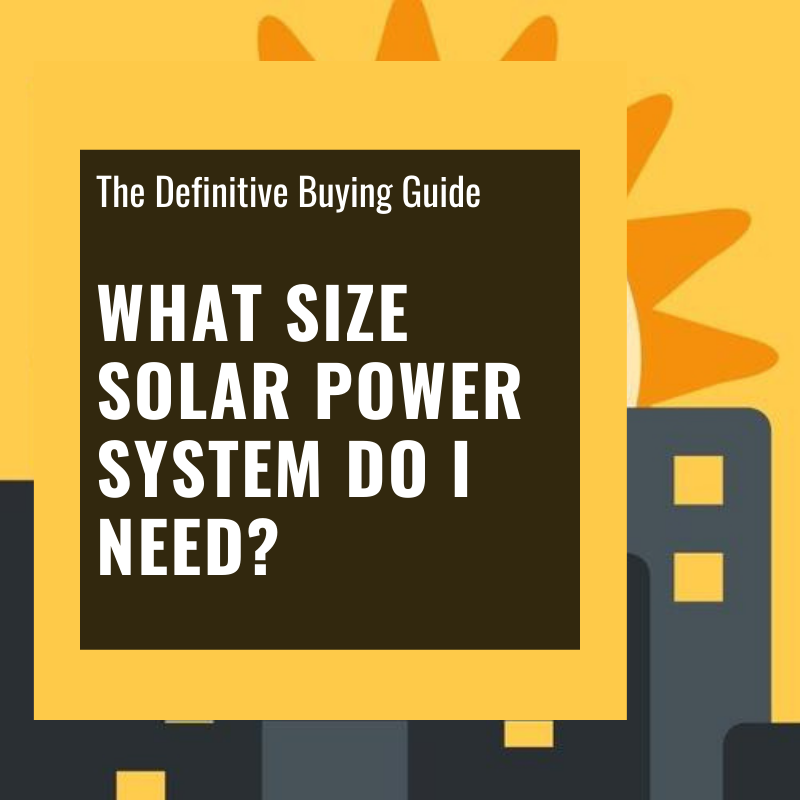# What Size Solar System Do I Need? The Definitive Solar Sizing Guide [DIY Solar Course Part 6/6]## Browse Popular Products

Sales & PromotionsSee our 2021 Holiday Specials
here!

When it comes to figuring out what size solar system you need, there are a couple simple calculations we’ll want to keep in mind. This is a super basic example, however you can always substitute our numbers for yours.

The first thing you’ll want to figure out is how many watts per day you’ll be using and for how long. We have a handy load calculator you can use for this here, but first let’s take a look at our example.

Let’s say you want to power a 150 watt LCD TV for 5 hours per day.

## Calculating how many batteries we need

We typically start with figuring out how much power we’ll need to store in order to handle our loads aka what size battery bank we’ll need. In order to calculate this, we first need to multiply our required watts by hours per day we’ll be using those watts. So in this example, the calculation is as follows:

150 watts x 5 hours per day = 750 watt hours (wh)

So with this, we see that we need to store at least 750 watt hours in order to meet our needs. However, we also need to factor in some backup time for when the sun isn’t shining or the weather is cloudy. Typically, we suggest factoring in 3-5 days of backup power depending on your location. From here, we’ll take the watt hours from our previous calculation and multiply it by 3 (using 3 days of backup).

750 wh x 3 = 2,250 wh

Now we know we’ll need at least a 2,250 wh battery bank. Let’s say you were going to go with Lion Energy’s 12 volt UT1200 as an example here, we would need 2 of these batteries in order to meet our 2,250 wh needs (2 x 1200 = 2,400 wh total). It’s always smart to be on the bigger side of things when it comes to storage since we never know what type of conditions we might run into. That being said, depending on what type of weather conditions you’re in, if you’re getting lots of sun, you could technically run off of one battery while keeping it fully charged by going with a much bigger array to offset the smaller battery.

## Calculating how many solar panels we need

Now that we know what size battery bank we need, it’s time to figure out how many solar panels (watts) we need to charge the battery bank in a reasonable amount of time. We always suggest running this calculation under the assumption we want to be able to fully recharge our battery bank everyday so that at night time our batteries are topped off. Using the standard 5 good sun hours per day, we’ll want to divide our battery bank size by 5.

2,400/5 = 480W

This means that we would need a minimum of a 480 watt solar array in order to charge this battery bank in one day with great conditions. Since panels are one of the most affordable parts of a system and we never know what type of sun conditions we’ll get, we always recommend going with slightly more, so in this case, 600 watts would be smart. This means we would need 6 x 100 watt solar panels or 2 x 300 watt panels.

## Calculating what size of solar charge controller we need

Now that we know what size of battery bank we need as well as the size of solar array we need to charge the battery bank in a day, it’s time to figure out what size solar charge controller we need. The charge controller regulates the voltage and current coming from the solar panels and going into the batteries.

To do this, we’ll need to divide the size of our solar array by the voltage of our batteries which then gives us the amps. So in this case, we’re using 12-volt Lion Energy batteries and we have a 600-watt solar array.

600 watts/12 volts = 50A

Again, it’s always smart to have a slightly bigger charge controllers in order to avoid overheating and charging issues, so for this example, we would suggest going with a 60A charge controller such as this one here.

### Final thoughts

Since watts/volts = amps, it’s important to note that the more voltage you have on your batteries, the more money you’ll generally save on your charge controller. For example, a 24 volt battery bank would only require a 25 or 30 amp charge controller since using our example from above 600/24 = 25 and the smaller the charge controller, the less expensive they are.

There’s always tons to consider when it comes to sizing your solar system but these calculations should give you a nice starting point for calculating what size of battery bank you’ll need, how many watts (panels) it will take to charge your battery bank and what size charge controller you’ll need to regulate the system.

From here there’s still lots left to consider but hopefully this helps! Let us know in the comments what size system you’re going with and how you ran your calculations.

Did you find this guide helpful? then you might also be interested in our blogs:

## Compare products

{"one"=>"Select 2 or 3 items to compare", "other"=>"{{ count }} of 3 items selected"}

Select first item to compare

Select second item to compare

Select third item to compare